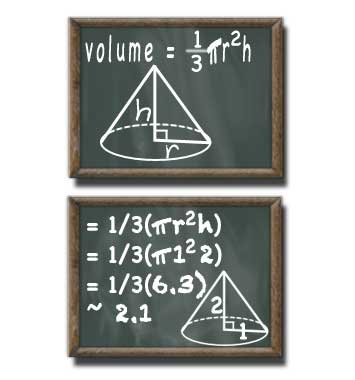# Cone Volume

### Kyle's Converter > Calculators > Geometry > Cone Volume

#### Cone Volume Calculation:

• ` r =`

Radius of the circular base of the cone

• ` h =`

Height of the cone from the center of the circular base to the tip

• ` V =`

Volume of the cone in cubic units

• `    `

Choose the number of decimals to show in your answer. This is also known as significant figures. Select an appropriate amount of significant figures based on the precision of the input numbers.

#### Cone Volume Formula

If a cone has a flat bottom, meaning the height and radius meet at right angles, then this formula can be used to find of volume ('V') of that cone (also know as a right circular cone):

V = 1/3(PI*r2h)Volume of a Cone Formula and Chalkboard Example

### Cone Volume Variables

To calculate the volume of the cone we must know the radius at the base and the height. The volume of the cone will be cubic units of whatever the radius and height have been measured in.

Radius of the circular base of the cone.
Height (h)
Height of the cone from the center of the circular base to the tip.
Volume (V)
Volume of the cone in cubic units.

### Cone Volume Solution

Cone volume is calculated from the radius and height. A cone is a circle that is pulled out along a third dimension to a single point. First calculate the area of the circle then multiply by one-third the height.

In plain english the volume of a cone can be calculated by taking one-third of the result of the radius squared, multiplied by the height, multiplied by the mathematical constant pi.

Here is a step-by-step case that illustrates how to find to volume of a cone with a radius of 2 feet and a height of 3 feet. Note that in order to save space (and because pi cannot be determine precisely) a limited number of decimal places are used, the symbol ~ denotes that this answer is an approximation.

• V = 1/3(PI*r2h)
• The formula used to calculate the volume of a cone
• V = 1/3(PI*223)
• Substitute in the radius of two and height of three
• V = 1/3(PI*4*3)
• Calculate the exponent inside the brackets
• V = 1/3(37.69908)
• Multiply the values inside the brackets, using 3.14159 for Pi
• V = 12.56636
• Multiply the brackets by one-third, i.e. divide by three
• ~12.56636

A cone with a radius of two feet and a height of three feet will have a volume of about 12.6 feet.

### Cone Volume Example

What is the volume of a cone with a radius of 2 inches and a height of 4 inches?

• V = 1/3(PI*r2h)
• V = 1/3(PI*224)
• V = 1/3(3.14159*4*4)
• V = 1/3(50.26544)
• ~16.75514

A cone with a radius of two inches and a height of four inches has a volume of about 16.8 cubic inches, which we can convert to fluid ounces.

### Formula for Volume of a Cylinder

Check out the cylinder volume calculator. Can you predict the difference between the formula for the volume of a cylinder and volume of a cone?